# DAV Class 8 Maths Chapter 8 Brain Teasers Solutions

The DAV Class 8 Maths Solutions and DAV Class 8 Maths Chapter 8 Brain Teasers Solutions of Polynomials offer comprehensive answers to textbook questions.

## DAV Class 8 Maths Ch 8 Brain Teasers Solutions

Question 1A.
Tick (✓) the correct option.

(i) Value of p for which (x2 + 3x + p) divisible by (x – 2) is
(a) 10
(b) – 10
(c) 5
(d) – 5
Solution:
(b) – 10

Let us divide x2 + 3x + p by x – 2. We haveQuotient = x + 5
Remainder = p + 10
If x2 + 3x + p is completely divisible by x – 2, then
Remainder = 0
p + 10 = 0
p = – 10.

(ii) Quotient obtained when (x2 + 7x – 4) is divided by (x + 7) is
(a) x
(b) x- 4
(c) x + 4
(d) x + 2
Solution:
(a) xQuotient = x
Remainder = – 4

(iii) Polynomial which when divided by (- x2 + x – 1) gives a quotient (x – 2) and remainder 3 is
(a) x3 – x2 + 3x – 5
(b) – x3 + 3x2 – 3x + 5
(c) – x3 + 3x2 – 3x – 5
(d) x3 – 3x2 – 3x + 5
Solution:
(b) – x3 + 3x2 – 3x + 5

Dividend = Divisor × Quotient + Remainder
= (- x2 + x – 1) × (x – 2) + 3
= x(- x2 + x – 1) – 2 (- x2 + x – 1) + 3
= – x3 + x2 – x + 2x2 – 2x + 2 + 3
= – x3 + 3x2 – 3x + 5

(iv) What should be added to the polynomial x2 – 5x + 4, so that (x – 3) is factor of the resulting polynomial?
(a) 1
(b) 2
(c) 4
(d) 5
Solution:
(b) 2

Let us divide x2 – 5x + 4 by (x – 3)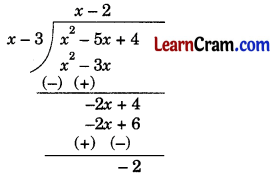As – 2 + 2 = 0, so to make (x – 3) a factor of x2 – 5x + 4, we should add (+ 2) to it.

(v) What should be subtracted from the polynomial x2 – 16x + 30, so that (x – 15) is factor of the resulting polynomial?
(a) 30
(b) 14
(c) 15
(d) 16
Solution:
(c) 15

Let us divide x2 – 16x + 30 by (x – 15)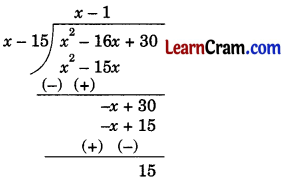As 15 – 15 = 0, so to make (x – 15) a factor of x2 – 16x + 30, we should subtract 15 from it.

Question 1B.

(i) Find the difference between the quotient and remainder when (x3 – 6x2 + 11x – 6) is divided by (x + 1).
(ii) If (x – 3) is a factor of the polynomial (2x2 + x + k), find k.
(iii) Can (x – 1) be the remainder on division of a polynomial p(x) by (x + 3). Justify your answer.
(iv) Find the remainder when (x3 + x – 4x2 + 6) is divided by (x + 2). What do you conclude whether (x + 2) is a factor of (x3 + x – 4x2 + 6) or not?
(v) If (8y + 8y2 + 7) is divided by (1 – y), then find the remainder.
Solution:
(i) Divide x3 – 6x2 + 11x – 6 by x + 1, as shown: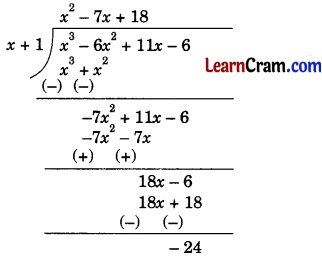Quotient = x2 – 7x + 18
Remainder = – 24
Difference of quotient and remainder = (x2 – 7x + 18) – (- 24)
= x2 – 7x + 18 + 24
= x2 – 7x + 42

(it) Dividing 2x2 + x + k by x – 3, we get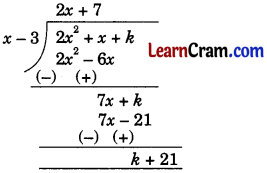Remainder = k + 21
If (x – 3) is a factor of (2x2 + x + k), then Remainder = 0
⇒ k + 21 = 0
⇒ k = – 21.

(iii) We see that degree of both (x + 3) and (x -1) is the same, i.e., 1. We know that degree of remainder must be less than that of divisor. So, (x – 1) can’t be the remainder on division of a polynomial p(x) by (x + 3).

(iv) x3 + x – 4x2 + 6 = x3 – 4x2 + x + 6.
Now, dividing x3 – 4x2 + x + 6 by x + 2, we get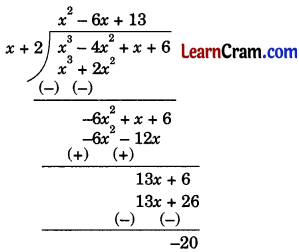Remainder = – 20
We conclude that (x + 2) is not a factor of x3 – 4x2 + x + 6 as remainder ≠ 0.

(v) Dividend = 8y + 8y2 + 7 – 8y – 16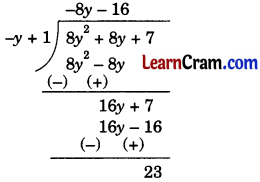Dividend in standard form = 8y2 + 8y – 16
Divisor = 1 – y = – y + 1
Quotient = – 8y – 16
Remainder = 23.

Question 2.
Divide:
(i) – 39x4 by $$\sqrt{13}$$ x2
(ii) $$\sqrt{125}$$y2 by 5y2
(iii) 49p3 by – 7$$\sqrt{7}$$ p2
(iv) 14z6 by $$\frac{14}{3}$$ z3
(v) 6x3 – 4x2 + 8x by $$\frac{2}{3}$$ x
(vi) y2 – 5y + 1 by $$\frac{-1}{3}$$ y
(vii) 18a3 – 12a2 + 9a by 3a
Solution:
(i) $$\frac{-39 x^4}{\sqrt{13} x^2}$$ = $$\frac{-39 \times \sqrt{13}}{\sqrt{13} \sqrt{13}}$$ x4 – 2
= $$\frac{-39 \sqrt{13} x^2}{13}$$
= – 3$$\sqrt{13}$$ x2

(ii) $$\frac{\sqrt{125} y^2}{5 y^2}=\frac{\sqrt{5 \times 5 \times 5}}{5} \cdot \frac{y^2}{y^2}$$
= $$\frac{5 \sqrt{5}}{5}$$
= $$\sqrt{5}$$

(iii) $$\frac{49 p^3}{-7 \sqrt{7} p^2}=\frac{7 \times 7}{-7 \sqrt{7}} p^{3-2}$$
= $$\frac{7 \times \sqrt{7}}{-\sqrt{7} \sqrt{7}}$$ p
= – $$\sqrt{7}$$ p

(iv) $$\frac{14 z^6}{\frac{14}{3} z^3}$$ = $$\frac{14 \times 3}{14}$$ . z6 – 3
= 3z3

(v) $$\frac{6 x^3-4 x^2+8 x}{\frac{2}{3} x}$$ = $$\frac{6 x^3}{\frac{2}{3} x}-\frac{4 x^2}{\frac{2}{3} x}+\frac{8 x}{\frac{2}{3} x}$$
= $$\frac{6 \times 3}{2} x^{3-1}-\frac{3 \times 4}{2} \cdot x^{2-1}+\frac{8 \times 3}{2}$$
= 9x2 – 6x + 12

(vi) $$\frac{y^2-5 y+1}{\frac{-1}{3} y}$$ = $$\frac{y^2}{\frac{-1}{3} y}-\frac{5 y}{\frac{-1}{3} y}+\frac{1}{\frac{-1}{3} y}$$
= – 3y2-1 + 5 3y1-1 – $$\frac{3}{y}$$
= – 3y + 15 – $$\frac{3}{y}$$

(vii)  = 
= 6a

Question 3.
(i) 4x2 + 7x – 11 by 2x – 4
(ii) 125 – 225x + 135x2 – 27x3 by 5 – 3x
(iii) y3 + 5y2 + 12y + 9 by y + 2
(iv) 8p3 – 729 – 108p2 + 486p by 2p – 9
(v) q4 + 3q2 – 4 by q2 – 1
(vi) p4 + $$\frac{63}{4}$$ p2 – 5 by p2 – $$\frac{1}{4}$$
Solution:
(i) 4x2 + 7x – 11 ÷ 2x – 4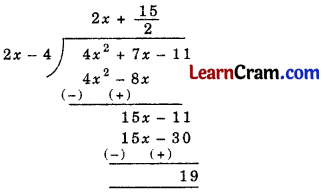Quotient = 2x + $$\frac{15}{2}$$
and Remainder = 19

Check:
Dividend = Divisor × Quotient + Remainder
4x2 + 7x – 11 = (2x – 4) (2x + $$\frac{15}{2}$$) + 19
= 2x (2x + $$\frac{15}{2}$$) – 4 (2x + $$\frac{15}{2}$$) + 19
= 4x2 + 15x – 8x – 30 + 19
= 4x2 + 7x – 11
L.H.S. = R.H.S.
Hence verified.

(ii) Let us write the dividend and the divisor in standard form.
– 27x3 + 135x2 – 225x + 125 and – 3x + 5Quotient = 9x2 – 30x + 25
and Remainder = 0

Check:
Dividend = Divisor × Quotient + Remainder
– 27x3 + 135x2 – 225x + 125 = (- 3x + 5) (9x2 – 30x + 25) + 0
= – 3x (9x2 – 30x + 25) + 5 (9x2 – 30x + 25)
= – 27x3 +90x2 – 75x + 45x2 – 150x + 125
= – 27x3 + 135x2 – 225x + 125
L.H.S. = R.H.S.
Hence verified.

(iii)Quotient = y2 + 3y + 6
and Remainder = – 3

Check:
Dividend = Divisor × Quotient + Remainder
y3 + 5y2 + 12y + 9 = (y + 2) x (y2 + 3y + 6) + (- 3)
= y (y2 + 3y + 6) + 2(y2 + 3y + 6) – 3
= y3 + 3y2 + 6y + 2y2 + 6y + 12 – 3
= y3 + 5y2 + 12y + 9
L.H.S. = R.H.S.
Hence verified.

(iv) Let us write the dividend in standard form.
8p3 – 108p2 + 486p – 729Check:
Dividend = Divisor × Quotient + Remainder
8p3 – 108p2 + 486p – 729 = (2p – 9) × (4p2 – 36p + 81) + 0
= 2p (4p2 – 36p + 81) – 9 (4p2 – 36p + 81)
= 8p3 – 72p2 + 162p – 36p2 + 324p – 729
= 8p3 – 108p2 + 486p – 729
L.H.S. = R.H.S. Hence verified.

(v)Quotient = q2 + 4
and Remainder = 0

Check:
Dividend = Divisor × Quotient + Remainder
q4 + 3q2 – 4 = (q2 – 1) (q2 + 4) + 0
= q2 (q2 + 4) – 1 (q2 + 4)
= q4 + 4q2 – q2 – 4
= q4 + 3q2 – 4
L.H.S. = R.H.S.
Hence verified.

(vi)Quotient = p2 + 16
and Remainder = – 1

Check:
Dividend = Divisor × Quotient + Remainder
p4 + $$\frac{63}{4}$$ p2 – 5 = (p2 – $$\frac{1}{4}$$) (p2 + 16) (- 1)
= p2 (p2 + 16) – $$\frac{1}{4}$$ (p2 + 16) – 1
= p4 + 16p2 – $$\frac{1}{4}$$ p2 – 4 – 1
= p4 + $$\frac{63}{4}$$ p2 – 5
L.H.S. = R.H.S.
Hence verified.

Question 4.
Find whether or not, the first polynomial is a factor of the second polynomial.
(i) 3x + 2, 3x4 + 5x3 – x2 + 13x + 10
(ii) x2 + 1, x4 – 3x3 – 4x2 + 3x + 2
Solution:
(i)Quotient = x3 + x2 – x + 5
and Remainder = 0
∴ 3x + 2 is the factor of 3x4 + 5x2 – x2 + 13x + 10.

(ii)Quotient = (x2 – 3x – 5)
and Remainder = (6x + 7)
∴ (x2 + 1) is not the factor of x4 – 3x3 – 4x2 + 3x + 2.

### DAV Class 8 Maths Chapter 8 HOTS

Question 1.
The sum of the remainders obtained when (x3 + (k + 8) x + k) is divided by (x – 2) or when it is divided by (x + 1) is zero. Find the value of k.
Sol.
x3 + (k + 8) x + k = x3 + kx + 8x + k

Case I:Case II:According to question,
Remainder of case I + Remainder of case II = 0
3k + 24 + (- 9) = 0
⇒ 3k + 15 = 0
⇒ k = – 5

Question 1.
State whether the given expression are polynomial or not. If not give reason.

(i) √3x + 5√x + 3
(ii) $$\frac{3}{2}$$ x3 + 1
(iii) √5x$$\frac{5}{2}$$ – √2x$$\frac{3}{2}$$+ √x
(iv) x + $$\frac{1}{x}$$ – 3
(v) 5x – $$\frac{2}{x}$$ + $$\frac{3}{x^2}$$ – 5
(vi) p2 + p – √p
Solution:
(i) √3x + 5√x + 3
The given expression is not a polynomial as the power of x is a rational number, i.e. $$\frac{1}{2}$$

(ii) $$\frac{3}{2}$$ x3 + 1
The given expression is a polynomial.

(iii) √5x$$\frac{5}{2}$$ – √2x$$\frac{3}{2}$$+ √x
The given expression is not a polynomial as the degree of the polynomial is rational.

(iv) 5x – $$\frac{2}{x}$$ + $$\frac{3}{x^2}$$ – 5
The given expression is not a polynomial as the power of x is an integer.

(v) p2 + p – √p
The given expression is not a polynomial as the power of x is a negative integer.

Question 2.
Divide 5x4 – 3x3 + 2x2 + 6x – 11 by 5x – 1. Find the remainder and the quotient then verify the answer.
Solution:Quotient = x3 – $$\frac{2}{5} x^2+\frac{8}{25} x+\frac{158}{125}$$
and Remainder = $$\frac{-1217}{125}$$

Verification:
Dividend = Divisor × Quotient + Remainder
5x4 – 3x3 + 2x2 + 6x – 11 = (5x – 1) × $$\left(x^3-\frac{2}{5} x^2+\frac{8}{25} x+\frac{158}{125}\right)-\frac{1217}{125}$$
= 5x $$\left(x^3-\frac{2}{5} x^2+\frac{8}{25} x+\frac{158}{125}\right)$$ – 1 $$\left(x^3-\frac{2}{5} x^2+\frac{8}{25} x+\frac{158}{125}\right)$$ – $$\frac{1217}{125}$$
= 5x4 – 2x3 + $$\frac{8}{5} x^2+\frac{158}{25} x-x^3+\frac{2}{5} x^2-\frac{8}{25} x-\frac{158}{125}-\frac{1217}{125}$$
= 5x4 – 3x3 + 2x2 + 6x – 11
L.H.S. = R.H.S.
Hence verified.

Question 3.
Divide x6 – 1 by x – 1 and verify the answer.
Solution: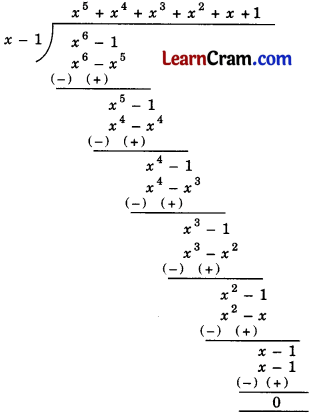Quotient = x5 + x4 + x3 + x2 + x + 1
and Remainder = 0

Verification:
Dividend = Divisor × Quotient + Remainder
x6 – 1 = (x – 1) (x5 + x4 + x3 + x2 + x + 1 )+ 0
= x (x5 + x4 + x3 + x2 + x + 1 ) – 1 (x5 + x4 + x3 + x2 + x + 1 )
= x6 + x5 + x4 + x3 + x2 + x – x5 – x4 – x3 – x2 – x – 1
= x6 – 1
L.H.S. – R.H.S.
Hence verified.

Question 4.
Using division, show that (3x + 1) is a factor of 12x3 – 2x2 + x + 1.
Solution: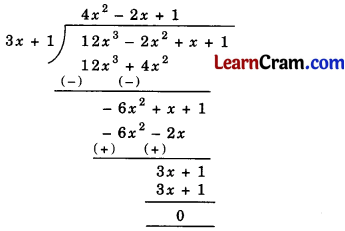Remainder = 0
Hence (3x + 1) is a factor of 12x3 + 2x2 + x + 1.

Question 5.
Using factor method, divide the following polynomial by the given binomial.
(i) x4 – 1 by x – 1
(ii) x2 – x – 42 by x – 7
Solution:
(i) $$\frac{x^4-1}{x-1}$$
⇒ $$\frac{\left(x^2+1\right)\left(x^2-1\right)}{(x-1)}$$
⇒ $$\frac{\left(x^2+1\right)(x+1)(x-1)}{(x-1)}$$
= (x2 + 1) (x + 1)
= x3 + x2 +x + 1
Hence the quotient is x3 + x2 + x + 1.

(ii) $$\frac{x^2-x-42}{x-7}$$
⇒ $$\frac{x^2-7 x+6 x-42}{x-7}$$
⇒ $$\frac{x(x-7)+6(x-7)}{(x-7)}$$
⇒ $$\frac{(x-7)(x+6)}{(x-7)}$$
= (x + 6)
Hence the quotient is (x + 6).

Question 6.
Divide 3x (5x2 + 10x3 + 5) – (x2 – 4 – 2x) by (- 3 + 5x).
Solution:
Dividend:
3x (5x2 + 10x3 + 5) – (x2 – 4 – 2x) = 15x3 + 30x4 + 15x – x2 + 4 + 2x
= 30x4 + 15x3 – x2 + 17x + 4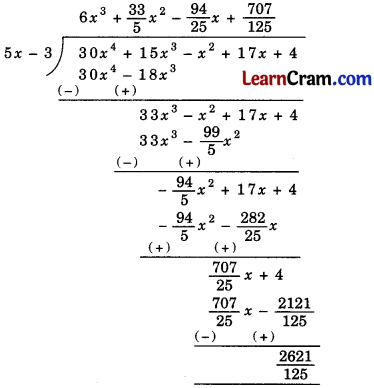Hence quotient = 6x3 + $$\frac{33}{5} x^2-\frac{94}{25} x+\frac{707}{125}$$
Remainder = $$\frac{2621}{125}$$

Question 7.
Divide: x4 + $$\frac{63}{4}$$ x2 – 5 by x2 – $$\frac{1}{4}$$ and check the answer.
Solution: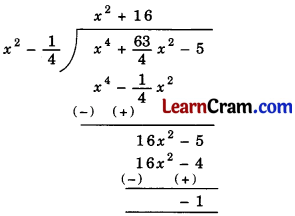Quotient = x2 + 16
and the remainder = – 1
Check:
Dividend = Divisor × Quotient + Remainder
x2 + 16 = (x2 – $$\frac{1}{4}$$) × (x2 + 16) – 1
= x2 (x2 + 16) – $$\frac{1}{4}$$ (x2 + 16) – 1
= x4 + 16x2 – $$\frac{1}{4}$$ x2 – 4 – 1
= x4 + $$\frac{63}{4}$$ x2 – 5
L.H.S. = R.H.S.
Hence verified.

Question 8.
Find the value of k if x2 – kx – 42 is divisible by x – 7.
Solution:If the given expression is completely divisible by (x – 7), then remainder = 0.
∴ – 7 (k – 7) – 42 = 0
Dividing both side by – 7,
(k – 7) + 6 = 0
∴ k = 1
Hence the value of k = 1.

Question 9.
Find the values of‘a’ and ‘b’ if the polynomial x3 + ax2 + bx – 12 is completely divisible by (x – 1) and (x + 3).
Solution:
Case I:Case II: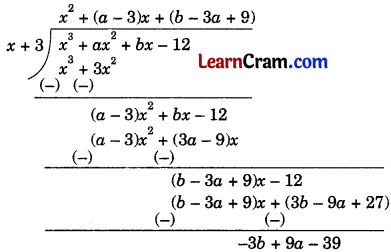If (x – 1) and (x + 3) are factors of x3 + ax2 + bx – 12, then remainders should be zero.

In Case I,
a + b – 11 = 0
⇒ a + b = 11 ………………(1)

In Case II,
9a – 36 – 39 = 0
⇒ 3a – b = 13
On solving equations (1) and (2),
a = 6 and b = 5 ……………..(2)

Question 10.
Find the value of ‘k’ if (x + k) is a factor of (x2 + 5x + 6).
Solution: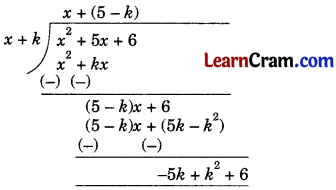If (x + k) is a factor of x2 + 5x + 6, then
Remainder = 0
k2 – 5k + 6 = 0
k2 – 3k – 2k + 6 = 0
= k (k – 3) – 2 (k – 3) = 0
(k – 3) (k – 2) = 0
If k – 3 = 0
⇒ k = 3
and if k – 2 = 0
⇒ k = 2.
Hence the values of k are 2 and 3.

Question 11.
Divide the following polynomials by a monomial.
(i) 8p3 + 16p2 + 14p + 1 by 4p
(ii) – 3x2 + √3x by √3x
Solution:
(i) $$\frac{-8 p^3+16 p^2+14 p+1}{4 p}$$ = $$\frac{-8 p^3}{4 p}+\frac{16 p^2}{4 p}+\frac{14 p}{4 p}+\frac{1}{4 p}$$
= – 2p2 + 4p + $$\frac{7}{2}+\frac{1}{4 p}$$

(ii) $$\frac{-3 x^2+\sqrt{3} x}{\sqrt{3} x}$$ = $$\frac{-3 x^2}{\sqrt{3} x}+\frac{\sqrt{3} x}{\sqrt{3} x}$$
= – √3x + 1

Question 12.
Divide the following by using long division method and check your answer.
(i) x3 – 3x2 + 3x – 1 by x – 1
(ii)12x + 10x3 + 8x4 + 15 by 5 + 4x
Solution:Check:
Dividend = Divisor × Quotient + Remainder
x3 – 3x2 + 3x – 1 = (x – 1) x (x2 – 2x + 1) + 0
= x (x2 – 2x + 1) – 1 (x2 – 2x + 1)
= x3 – 2x2 + x – x2 + 2x – 1
= x3 – 3x2 + 3x – 1
L.H.S. = R.H.S.
Hence verified.

(ii) Let us write the dividend and the divisor in standard form.
Dividend = 8x4 + 10x3 + 12x + 15
and Divisor = 4x + 5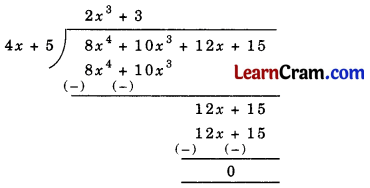Check:
Dividend = Divisor × Quotient + Remainder
8x4 + 10x3 + 12x + 15 = (4x + 5) × (2x3 + 3) + 0
= 4x (2x3 + 3) + 5 (2x3 + 3)
= 8x4 + 12x + 10x3 + 15
= 8x4 +10x3 + 12x + 15
L.H.S. = R.H.S.
Hence verified.

Question 13.
Check whether the second polynomial is a factor of first polynomial. Use long division method to check,
(i) 2y3 – 14y + 12; y + 3
(ii) 3x2 – 11x + 6; x – 3
Solution: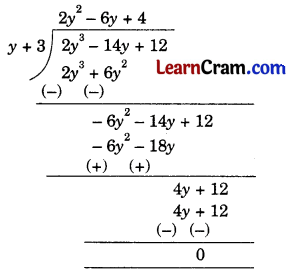Here, remainder = 0
Hence, y + 3 is a factor of 2y3 – 14y + 12.

(ii)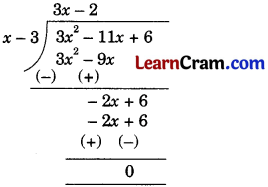Here, remainder = 0
Hence, x – 3 is a factor of 3x2 – 11x + 6.

Question 14.
Degree of the polynomial q10 + q6 – q4 + q8 is
(a) 4
(b) 6
(c) 10
(d) 8
Solution:
(c) 10
Degree of the given polynomial is 10.

Question 15.
Using division method, show that (3x + 1) is a factor of the polynomial 12x3 – 2x2 + x + 1.
Solution:Quotient = 4x2 – 2x + 1
and Remainder = 0
Hence (3x + 1) is a factor of the polynomial 12x3 – 2x2 + x + 1.

Question 16.
Divide: (y2 + 2y – 35) by (y + 7) using factor method.
Solution:
$$\frac{y^2+2 y-35}{y+7}$$ = $$\frac{y^2+7 y-5 y-35}{y+7}$$
= $$\frac{y(y+7)-5(y+7)}{y+7}$$
= $$\frac{(y+7)(y-5)}{y+7}$$
= y – 5
Hence Quotient = y – 5
and Remainder = 0

Question 17.
Find the quotient and remainder, when – 6x4 + 5x2 + 11x + 1 is divided by 2x2 + 1.
Solution: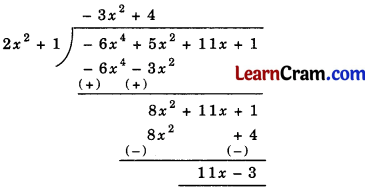Quotient = – 3x2 + 4
and the Remainder = 11x – 3

Question 18.
Divide (- 1 + x4) by (- 1 + x) and verify your answer.
Solution: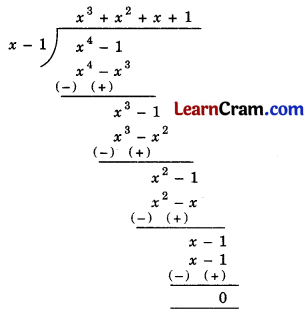Verification:
Dividend = Divisor × Quotient + Remainder
x4 – 1 = (x – 1) × (x3 + x2 + x + 1) + 0
= x (x3 + x2 + x + 1) – 1 (x3 + x2 + x + 1)
= x4 + x3 + x2 + x – x3 – x2 – x – 1
= x4 – 1
L.H.S. = R.H.S.
Hence verified.

Multiple Choice Questions:

Question 1.
If x – 1 is a factor of x2 – 2kx + 1, then the value of k is
(a) 0
(b) 2
(c) 1
(d) – 1
Solution:
(c) 1

Question 2.
If 6x2 – 13x + 6 is divisible by 2x – 3, then the remainder is
(a) 0
(b) 3x – 2
(c) 1
(d) None of these
Solution:
(a) 0

Question 3.
If 8x3 – 6x2 + 10x + 3 is completely divisible by (4x + 1), then the quotient is
(a) 2x2 + 2x – 3
(b) 2x2 – 3x + 3
(c) 2x2 – 2x – 3
(d) 2x2 – 2x + 3
Solution:
(d) 2x2 – 2x + 3

Question 4.
The value of k for which x6 – k is completely divisible by x – 1 is
(a) – 1
(b) 0
(c) – 2
(d) 1
Solution:
(d) 1

Question 5.
If x4 – a4 is divisible by x2 + a2, then the quotient is
(a) x2 – a2
(b) x – a
(c) x + a
(d) 0
Solution:
(a) x2 – a2